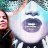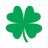# Polynomial Regression Channel (PRC)

C

#### carneyse71

##### New member
VIP
Looking for a PRC for TOS Can anyone give some insight for this?#### RobertPayne

##### Member
If you just need a 2nd degree polynomial, that can easily be programmed following the equations provided on this website: https://www.easycalculation.com/statistics/learn-quadratic-regression.php

If you need a higher order polynomial, that will require solving matrices and is much more involved. I have one that plots up to 6th degree polynomials available on my website. So, if you want something more advanced, visit my site.Last edited:
C

#### carneyse71

##### New member
VIP
@RobertPayne thank you much appreciated.#### markos

##### Well-known member
VIP
@RobertPayne Thank you for sharing that. Your help is appreciated.#### J007RMC

##### Well-known member
2019 Donor
VIP
hello Robert how are ya doing. Quick question for settings over 3rd degree such as time frames?

P

#### Piper2808t

##### Member
2019 Donor
VIP
does this repaint?#### BenTen

Staff
VIP
@Piper2808t If I'm not mistaken, anything with ZigZag or Regression will repaint.

•Piper2808t
I

#### ItaloFabianOfficial

##### New member
@RobertPayne Would it be possible to provide the content of your site again containing the polynomial regression made by you?

H

#### hockeycoachdoug

##### Member
2019 Donor
VIP
Does anyone have the TOS code to plot this? It would be a great first sort for prices near one of the extremes, then use the Ultimate Breakout indicator on a lower time frame and only take the buys when prices near bottom deviation and slope is positive and sells when at top deviation and negative slope.

C

#### codydog

##### Active member
@hockeycoachdoug -- if you look at the onenote which tos' thinkscript group has, and search for growex, I think you'll find some poly stuff

•hockeycoachdoug
H

#### hockeycoachdoug

##### Member
2019 Donor
VIP
@codydog- thanks for pointing me in a direction, I just couldn't find the direction you were pointing. I tried searching "growex" and "polynomial" on the onenote site I was familiar with with no luck. Can you point me a bit more specifically. Thanks in advance for your help.

Also, @RobertPayne- any chance of a discount to usethinkscript members for your indicators for sale on your site?

O

#### OBW

##### New member
Also, @RobertPayne- any chance of a discount to usethinkscript members for your indicators for sale on your site?

@RobertPayne posted a discount code for all usethinkscript members a few months ago and was promptly permanently banned by the admin.

•hockeycoachdoug
H

2019 Donor
VIP
Ooops, sorry

C

#### codydog

##### Active member
@hockeycoachdoug - they are putting up his stuff with a "xxx_VM" instead of growex,

no idea why his stuff isnt posted by author as he's done some good stuff

•hockeycoachdoug
H

#### hockeycoachdoug

##### Member
2019 Donor
VIP
codydog- I hate to seem stupid, but I searched for growex, xxx_VM, polynomial and still nothing shows. Am I looking in the right OneNote? I am using this one- ThinkScript Cloud

O

#### OBW

##### New member
•hockeycoachdoug
H

#### hockeycoachdoug

##### Member
2019 Donor
VIP
Thank you OBW and codydog for your help#### markos

##### Well-known member
VIP
@hockeycoachdoug
See if this is what you're looking for. I found it in the Thinkscript Cloud.
Code:
``````# Quadratic Regression
# Robert Payne
# funwiththinkscript.com

input length = 20;
def n = length;
def bn = BarNumber();
def lastBar = HighestAll(if IsNaN(close) then 0 else bn);
def x = bn;
def y = close;

# calculate summation values

def startBar = lastBar - (n - 1);
def sumX = if bn < startBar then 0 else x + sumX;
def sumY = if bn < startBar then 0 else y + sumY;
def sumX2 = if bn < startBar then 0 else Power(x, 2) + sumX2;
def sumX3 = if bn < startBar then 0 else Power(x, 3) + sumX3;
def sumX4 = if bn < startBar then 0 else Power(x, 4) + sumX4;
def sumXY = if bn < startBar then 0 else x * y + sumXY;
def sumX2Y = if bn < startBar then 0 else Power(x, 2) * y + sumX2Y;

# intermediary calculations

def xx = sumX2 - Power(sumX, 2) / n;
def xy = sumXY - (sumX * sumY / n);
def xx2 = sumX3 - (sumX2 * sumX / n);
def x2y = sumX2Y - (sumX2 * sumY / n);
def x2x2 = sumX4 - (Power(sumX2, 2) / n);

# calculate coefficients for the quadratic equation

def a0 = (x2y * xx - xy * xx2) / (xx * x2x2 - Power(xx2, 2));
def b0 = (xy * x2x2 - x2y * xx2) / (xx * x2x2 - Power(xx2, 2));
def c0 = sumY / n - b0 * sumX / n - a0 * sumX2 / n;

# for a, b, and c use the final value on the last bar of the chart for all calculations

def a = GetValue(a0, bn - lastBar);
def b = GetValue(b0, bn - lastBar);
def c = GetValue(c0, bn - lastBar);

# calculate and plot the regression curve

plot theCurve = if bn < startBar then Double.NaN else a * Power(x, 2) + b * x + c;
#####``````

If it works, please post a picture here!

•hockeycoachdoug
H

#### hockeycoachdoug

##### Member
2019 Donor
VIP
Thank you MarkosLinear Regression Slope Angle Plot Questions 0Linear Regression Trading Strategy for ThinkorSwim Strategies 14